Sedimentation Analysis - Stokes LawSmall particles of particular size range in liquid suspension have a tendency to settle down due to multiple type of forces acting on them. This behaviour of particles is known as sedimentation.

In soil mechanics we use this property of small particles to analyze the particle size distribution. Particle size distribution is the method of separation of soil sample into different fractions based on their particles sizes.

Soils with particle size greater than 75 micron are analyzed by Sieve analysis method using sieves of different sizes.

Particles of size smaller than 75 micron cannot be sieved because smaller particles carry charges on their surface and have tendency to stick to each other and other particles, even to the sieves or to the experimenter’s hand. So handling such kind of soils would be very difficult.

So to analyze soils containing these particles we use the method sedimentation analysis.

This method is based on the Stokes law.

According to this law a small particle in a liquid suspension tries to settle down due to its own weight under the action of gravity. And because of acceleration due to gravity its downward velocity keeps on increasing.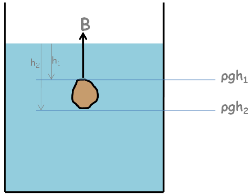But two forces,

One is Buoyant force, which acts upwards and acts due to the pressure difference on the body inside the liquid.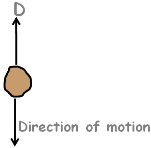second is drag force, which is a resistive force and acts opposite to the direction of motion of the body.

These two forces starts acting on the particle in the opposite direction of particle’s motion and start retarding the particle until it acquires the equilibrium condition. And because of which particle’s velocity becomes constant. That is, now particle falls with a constant velocity, which is called its terminal velocity.The expression for terminal velocity can be obtained by writing equation of equilibrium for this falling particle in fluid.

Particle’s weight W downward, buoyant force B and drag force D upwards.

So equilibrium equation will be W equal to B plus D.

W = B + D

Weight can be written as volume of particle multiplied by its unit weight gamma s.

Buoyant force will be weight of liquid, in our case that liquid is water, this particle has displaced which is volume of particle multiplied by the unit weight of water it displaces.

Drag force on a small spherical particle moving through a viscous fluid is given by 6 pie eta are wee.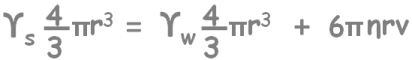Eta is the dynamic viscosity of soil suspension

Dynamic viscosity is also represented as letter mu.

Ys is unit weight of soil particle

And Yw is unit weight of water

Solving this equation and after a little rearrangement we get the terminal velocity as this.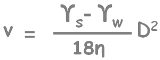Remember this equation as equation number 1.

In this equation these quantities are constant for a particular soil and water and viscosity is constant for a particular temperature.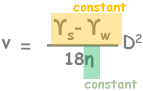So we can see the relationship between settling terminal velocity of the particle and its diameter.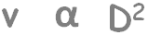We can see that larger the diameter of a particle, higher its speed will be and smaller the particle slower it will settle to the bottom.

Now by simple equation of motion we know that velocity of any particle which falls through a height of He centimeter in time t mins, would be He upon t. Here substitute the value of velocity from the equation number 1 and we get this equation.remeber this as equation number 2. Here these quantities are constant,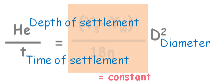So we observe a relationship between diameter of a soil particle and its time of settlement to the depth He. By this we can calculate the time required t by any soil particle of diameter D to settle down to a depth of He.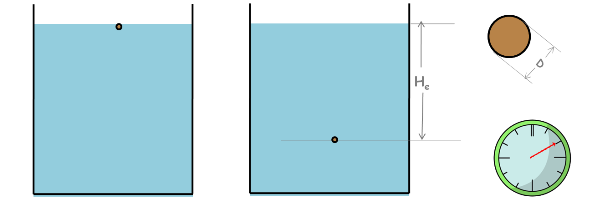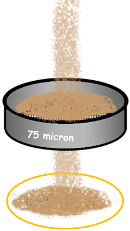That’s ok, but how do we carry out particle size analysis with this method?

To start with let’s first prepare the soil suspension for sedimentation analysis.

We take about 50 gm of oven dried soil sample which passed through 75 micron sieve. Put it into a vessel and mix about 100 ml of a dispersing agent.We need to mix dispersing agent because fine soil particles tends to stick to each other and in the liquid suspension they form bigger flocs. These flocs behave like a single particle and according to above established equation number 1, they settle with greater speed than the individual particles should settle. Hence true sedimentation results cannot be obtained.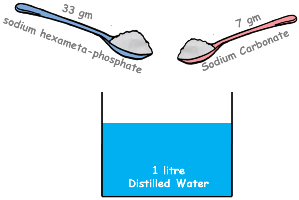There is also a method given for the preparation of dispersing agent. We prepare it by mixing 33 gm of sodium hexameta-phosphate and 7 gm of sodium carbonate to one litre of distilled water.

Back to our sample, after mixing the dispersing agent the sample is stirred with a mechanical stirrer for few minutes.

Then sample is transferred to a measuring jar and distilled water is added to it to make the suspension volume 1000 ml.

By placing a hand over the jar make the jar upside down a few times so as to ensure complete mixing.Here we assume that our suspension is completely mixed and is homogeneous. That means we assume that all the soil particles of different sizes are uniformly distributed throughout the suspension and concentration of particles of different sizes is the same at all depths. This means the number of different sized particles at any depth is the same.

For clarity let’s take fewer particles and show all the particles of different sizes separated.

As per the equation number 1 we can know particles of larger diameter will have higher settling velocity and will settle earlier then small sized particles.

At the beginning of the sedimentation, amount of particles at any layer of the suspension will be same. But as time proceeds, particles start settling down and amount of particles of different layers become different.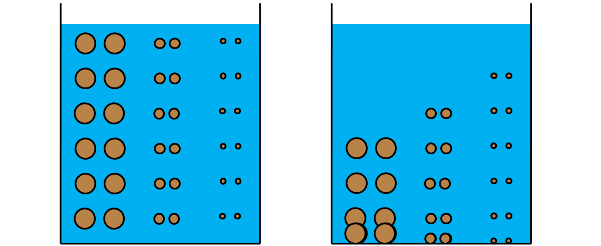By equation number 2 we know that in time t a particle of diameter D would have settled for He depth, which means above this depth He, only particles of size smaller than D will be present, because He is the depth from the surface and we are considering the particle of size D which has traveled the maximum distance so we can be sure that no particle of this size will be present above this layer.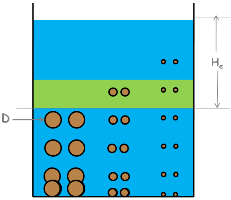So if we take a sample from the depth He all particles smaller than D will be present in this sample and in the same concentration as were in the beginning because of being the same settling velocity of similar sized particles. That is, two particles will leave the layer and two particles will arrive in the layer at the same time. So number of particles of particular size on the layer will remain the same.

As we know percentage finer is weight of sample with particles of size smaller than D divided by weight of the total soil sample with all the particles present in it.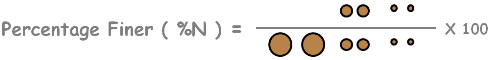By taking weight of highlighted layer at the beginning and after time t we can simply calculate percentage finer.

To determine the weight of soil particles on this layer we employ two methods

1. pipette method

2. hydrometer method.

In the pipette method the weight of solids is determined directly by collecting sample of soil suspension from a specified sampling depth using pipette.

In hydrometer method the weight of solids is calculated indirectly by reading the density of soil suspension on hydrometer.

There are some limitations of the Stokes law, and hence sedimentation analysis does not give correct values of the particle size analysis and the percentage finer. These limitations are eventually also the limitations of both pipette and hydrometer method.

1. Stokes law is based on the assumption that all the soil particles are spherical. But fine soils particles are never spherical and actually are flaky or needle shaped in reality.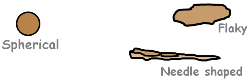2.specific gravity of solids for different particles is different, but we use its average value.3. Stokes law is applicable when fall of particle is in the liquid which has infinite extent, that is no boundary is present. But our suspension is in the jar and its walls affect the results to some extent as falling particles may collide with the wall.

4. we have also assumed that there is no interference on the movement of a particle by other surrounding particles. But practically there is a lot of interference. Particles may collide and may change their course and velocity.

However it has been shown that for suspension of 50 gm per litre or less particle to particle influence is negligible.

5. Sedimentation analysis cannot be used for particles of size larger than 0.2 mm because they may cause to generate turbulent conditions and stokes law is not applicable for that.

6. Sedimentation analysis is also not applicable for particles smaller than 0.2 micron because such small particles may set Brownian motion in the suspension and these particles do not settle as per stokes law.

Tags : Sedimentation, stokes law

Published on :2019-09-06